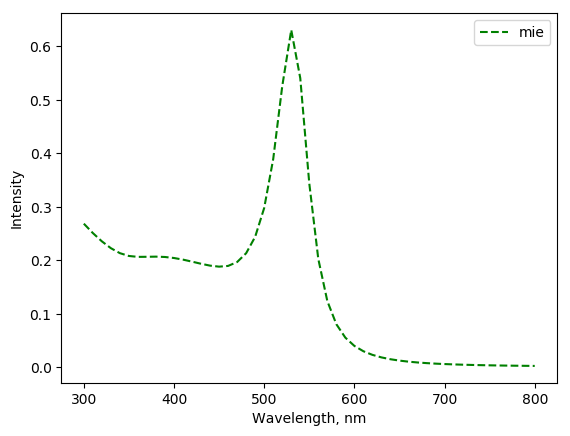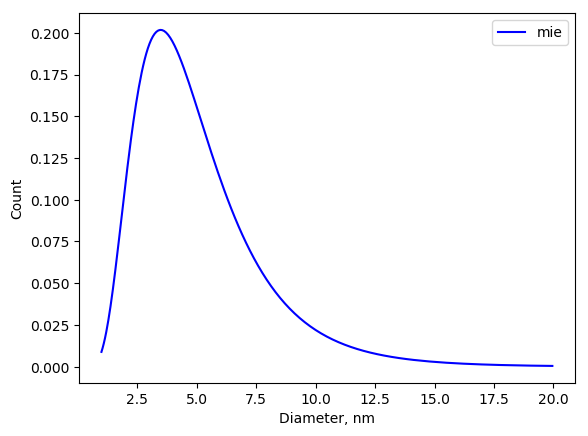# Simple functions and Mie theory¶

## Example¶

The example how to obtain contribution to the extinction from Log-Normally distributed spheres. Other contributions are evaluated in similar way.

from mstm_studio.contributions import MieLognormSpheres
from mstm_studio.alloy_AuAg import AlloyAuAg
import numpy as np

mie = MieLognormSpheres(name='mie', wavelengths=np.linspace(300,800,51))
mie.set_material(AlloyAuAg(x_Au=1), 1.5)  # golden sphere in glass

values = [1, 1.5, 0.5]  # scale, mu, sigma
fig, _ = mie.plot(values)
fig.savefig('mie_contrib.png', bbox_inches='tight')mie.MAX_DIAMETER_TO_PLOT = 20   # 100 nm is by default
fig, _ = mie.plot_distrib(values)
fig.savefig('mie_distrib.png', bbox_inches='tight')## Classes¶

Contributions to UV/vis extinction spectra other then obtained from MSTM.

class mstm_studio.contributions.Contribution(wavelengths=[], name='ExtraContrib')[source]

Abstract class to include contributions other then calculated by MSTM. All lightweight calculated contribtions (constant background, lorentz and guass peaks, Mie, etc.) should enhirit from it.

Parameters:

wavelengths: list or numpy array
wavelengths in nm
name: string
optional label
calculate(values)[source]

This method should be overriden in child classes.

Parameters:

values: list of control parameters

Return:

numpy array of contribution values at specified wavelengths
plot(values, fig=None, axs=None)[source]

plot contribution

Parameters:

values: list of parameters

fig: matplotlib figure

axs: matplotlib axes

Return:

filled/created fig and axs objects
set_wavelengths(wavelengths)[source]

Modify wavelengths

class mstm_studio.contributions.ConstantBackground(wavelengths=[], name='ExtraContrib')[source]

Constant background contribution $$f(\lambda) = bkg$$.

Parameters:

wavelengths: list or numpy array
wavelengths in nm
name: string
optional label
calculate(values)[source]

Parameters:

values: [bkg]

Return:

numpy array
class mstm_studio.contributions.LinearBackground(wavelengths=[], name='ExtraContrib')[source]

Two-parameter background $$f(\lambda) = a \cdot \lambda + b$$.

Parameters:

wavelengths: list or numpy array
wavelengths in nm
name: string
optional label
calculate(values)[source]

Parameters:

values: list of control parameters scale, mu and Gamma

Return:

numpy array
class mstm_studio.contributions.LorentzBackground(wavelengths=[], name='ExtraContrib')[source]

Lorentz peak in background. Peak center is fixed.

$L(\lambda) = \frac {scale} {(\lambda-center)^2 + \Gamma^2}$

Parameters:

wavelengths: list or numpy array
wavelengths in nm
name: string
optional label
calculate(values)[source]

This method should be overriden in child classes.

Parameters:

values: list of control parameters

Return:

numpy array of contribution values at specified wavelengths
class mstm_studio.contributions.LorentzPeak(wavelengths=[], name='ExtraContrib')[source]

Lorentz function

$L(\lambda) = \frac {scale} {(\lambda-\mu)^2 + \Gamma^2}$

Parameters:

wavelengths: list or numpy array
wavelengths in nm
name: string
optional label
calculate(values)[source]

Parameters:

values: list of control parameters scale, mu and Gamma

Return:

numpy array
class mstm_studio.contributions.GaussPeak(wavelengths=[], name='ExtraContrib')[source]

Gauss function

$G(\lambda) = scale \cdot \exp\left( - \frac{(\lambda-\mu)^2}{2\sigma^2} \right)$

Parameters:

wavelengths: list or numpy array
wavelengths in nm
name: string
optional label
calculate(values)[source]

Parameters:

values: list of control parameters scale, mu and sigma

Return:

numpy array
class mstm_studio.contributions.MieSingleSphere(wavelengths=[], name='ExtraContrib')[source]

Mie contribution from single sphere.

Details are widely discusses, see, for example [Kreibig_book1995]

Parameters:

wavelengths: list or numpy array
wavelengths in nm
name: string
optional label
calculate(values)[source]

Parameters:

values: list of control parameters scale, diameter

Return:

extinction efficiency array of Mie sphere
set_material(material, matrix=1.0)[source]

Define the material of sphere and environment

Parameters:

material: Material object
material of the sphere
matrix: float, string or Material object
material of the environment

Return:

True if properties were changed, False - otherwise.
class mstm_studio.contributions.MieLognormSpheres(wavelengths=[], name='ExtraContrib')[source]

Mie contribution from an ensemble of spheres with sizes distributed by Log-Normal law

Parameters:

wavelengths: list or numpy array
wavelengths in nm
name: string
optional label
calculate(values)[source]

Parameters:

values: list of control parameters scale, mu and sigma

Return:

Mie extinction efficiency of log-normally distributed spheres
lognorm(x, mu, sigma)[source]

The shape of Log-Normal distribution:

$LN(D) = \frac {1}{D \sigma \sqrt{2\pi}} \exp\left( - \frac{(\log(D)-\mu)^2}{2\sigma^2} \right)$
plot_distrib(values, fig=None, axs=None)[source]

Plot size distribution

Parameters:

values: list of control parameters

fig: matplotlib figure

axs: matplotlib axes

Return:

filled/created fig and axs objects
set_material(material, matrix=1.0)[source]

Define the material of sphere and environment

Parameters:

material: Material object
material of the sphere
matrix: float, string or Material object
material of the environment

Return:

True if properties were changed, False - otherwise.
class mstm_studio.contributions.MieLognormSpheresCached(wavelengths=[], name='ExtraContrib')[source]

Mie contribution from an ensemble of spheres with sizes distributed by Lognormal law.

Cached version - use it to speed-up fitting.

Parameters:

wavelengths: list or numpy array
wavelengths in nm
name: string
optional label
calculate(values)[source]

Parameters:

values: list of control parameters scale, mu and sigma

Return:

Mie extinction efficiency of log-normally distributed spheres
 [Kreibig_book1995] Kreibig, M. Vollmer, “Optical Properties of Metal Clusters” (1995) 553